As in everyday life, solutions of differing concentration are described as ‘strong’ and ‘weak’

Students will often see these terms as being synonymous with ‘concentrated’ and ‘dilute’. This activity is designed to diagnose whether students distinguish strength from concentration.

## Explaining acid strength

One definition of an acid is that it dissolves in water to give hydrogen ions (H+).

In fact the hydrogen ion (H+) will associate with a water molecule to form H3O+.

One way to write the equation for an acid ‘HA’ dissolving in water is:

HA + H2O(l) ⇌ H3O+(aq) + A(aq)

The A in HA does not stand for a particular element, but for the ‘acid radical’ part of the molecule. So, for example, in hydrochloric acid ‘HA’ would be HCl, and ‘A’ would be Cl, whilst in ethanoic acid ‘HA’ would be CH3COOH, and ‘A’ would be CH3COO

Acids (and alkalis) can be described as ‘strong’ or ‘weak’, and as ‘concentrated’ or ‘dilute’.

1. What is the difference between a strong acid and a weak acid?
2. What is the difference between a concentrated acid and a dilute acid?
3. If you could see the particles (molecules, ions etc) in an acidic solution, how would you decide whether it was a solution of a strong acid or a solution of a weak acid?

## Classifying acid solutions

One way to write the equation for an acid ‘HA’ dissolving in water is:

HA + H2 O(l) ⇌ H3 O+(aq) + A–(aq)

Below are some diagrams of acidic solutions.

Scientific diagrams are always simplifications designed to highlight some aspects of the system represented. The diagrams in this exercise show simplifications of real solutions.

For example, the concentration of acids varies over many orders of magnitude, and an accurate diagram of a very dilute solution would need to show many thousands of water molecules for each H+(aq) ion.

Only four types of particle are shown in these diagrams. The following key is used to distinguish between the different particles: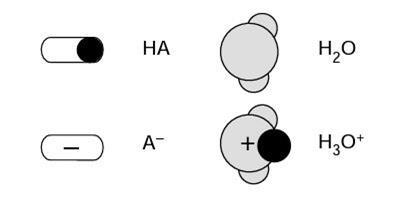The size (and shape) of acid molecules varies greatly, and they are often much larger than a water molecule.

Look carefully at the four diagrams on the below, and see if you can tell what the differences between them are meant to indicate.

## Diagram 1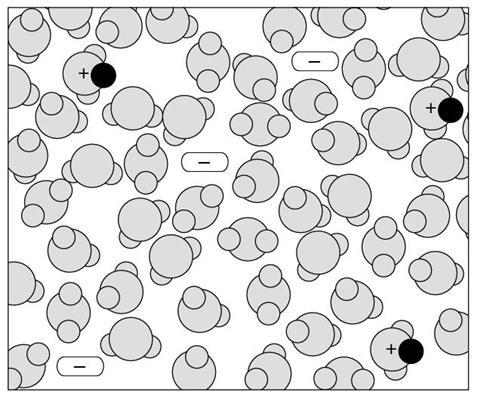1. What types of particles are shown in the solution represented in this diagram?
2. How would you describe this solution?

## Diagram 2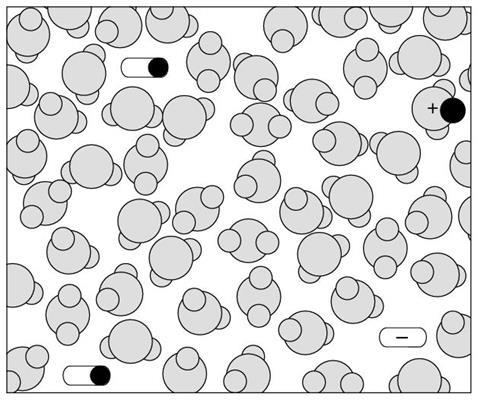1. What types of particles are shown in the solution represented in this diagram?
2. How would you describe this solution (compared to diagram 1)?

## Diagram 3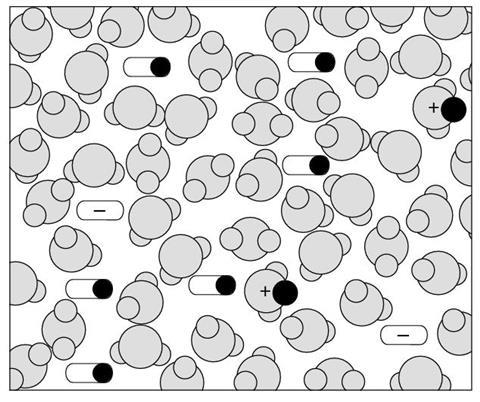1. What types of particles are shown in the solution represented in this diagram?
2. How would you describe this solution (compared to diagram 1 & 2)?

## Diagram 4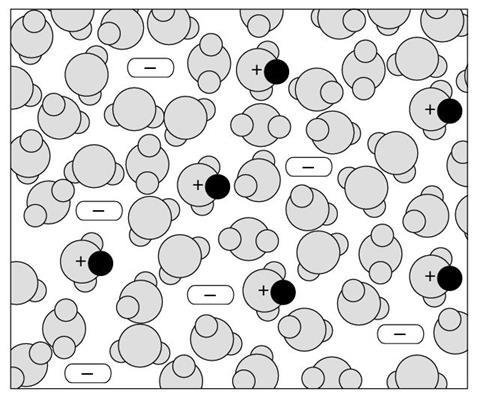1. What types of particles are shown in the solution represented in this diagram?
2. How would you describe this solution (compared to diagram 1, 2 & 3)?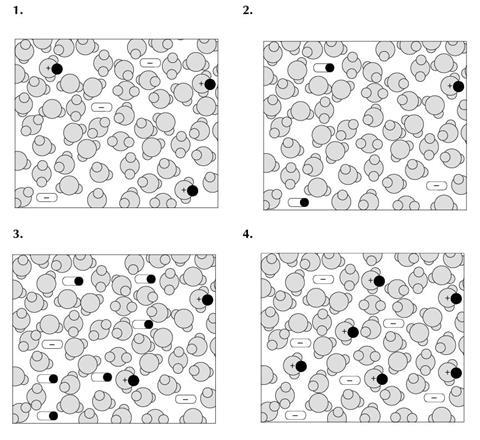1. The four diagrams you were asked to consider are reproduced in miniature above.

The diagrams are meant to represent a concentrated solution of a strong acid, a dilute solution of a strong acid, a concentrated solution of a weak acid and a dilute solution of a weak acid. Use the table below to show which diagram is meant to represent each of the four solutions – write the number of the appropriate diagram in each box.

Strong Weak
Concentrated
Dilute

### Explaining acid strength

1. In a strong acid all (or virtually all) of the molecules dissociate to give hydrated hydrogen ions and anions in solution. In a weak acid, only a proportion of the molecules dissociate to give hydrated hydrogen ions and anions in solution – so there are solvated molecules present as well as solvated ions.
2. A concentrated acid has a relatively large amount of solute dissolved in the solvent. A dilute acid has a relatively smaller amount of solute dissolved in the solvent.
3. In a solution of a strong acid there would be solvated ions present, but no (or only a very small proportion of) associated molecules present. In a solution of a weak acid there would be a significant proportion of molecules present in their original form, as well as some ions formed by dissociation of molecules.

### Classifying acid solutions

1. Water molecules, hydrated hydrogen ions (ie hydroxonium ions), anions (ie acid radicals) or H2O, H3O+, A.
2. The diagram shows a solution of a strong acid.
3. Water molecules, molecules of the acid, hydrated hydrogen ions (ie hydroxonium ions), anions (ie acid radicals), or H2O, HA, H3O+, A.
4. This diagram shows a solution of a weaker acid than that shown in diagram 1.
5. Water molecules, molecules of the acid, hydrated hydrogen ions (ie hydroxonium ions), anions (ie acid radicals) or H2O, HA, H3O+, A.
6. This diagram shows a weaker acid than that shown in diagram 1. This diagram shows a more concentrated acid than that shown in diagrams 1 and 2.
7. Water molecules, hydrated hydrogen ions (ie hydroxonium ions), anions (ie acid radicals), or H2O, H3O+, A.
8. This diagram shows an acid which is more concentrated than that shown in diagram 1 or 2. This diagram shows an acid which is stronger than that shown in diagrams 2 or 3.
Strong Weak
Concentrated  4  3
Dilute  1  2